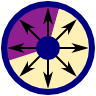The Coaxial Capacitor with Two Embedded DielectricsModern electronic devices are made of certain fundamental building blocks, for example capacitors. Understanding their properties is with respect to their geometrical parameters is of great importance particularly in electrical engineering. In this problem we will calculate the features of a coaxial capacitor that contains two different media.Problem Statement

A cylindrical (or coaxial) capacitor is made of two concentric metallic cylinders. Let the radius of the inner cylinder be $$r_{i}$$ and $$r_{o}$$ for the outer one. In-between the cylinders are two media with different relative permittivities $$\varepsilon_{1}$$ and $$\varepsilon_{2}$$. The two boundaries between these media may also be radial, see schematic on the right. Each medium has a certain filling fraction $$\alpha_{1}$$ and $$\alpha_{2}$$, respectively.

Calculate the capacitance $$C$$ per length $$l$$ for this device with respect to the given parameters.

Background

Microelectronics is a truly fascinating field. Let's take a close look at some motherboard which is the central part of a computer - the processor, graphics card etc. are connected to it:On the left we can see the cooler of the processor. Close to it there is a line of labeled pillars - electrolytic capacitors. These components are one of the main building blocks of electronic devices. Their inner structure is a little bit more complicated than that idealized coaxial capacitor we want to understand in the given problem. We will however be able to deduce some physical properties.